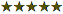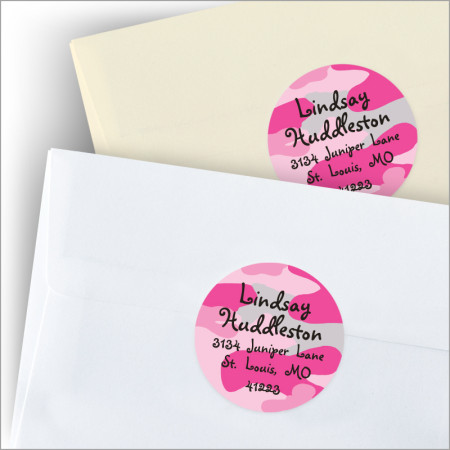# Pink Camo Round Stickers

Price per Set: \$?.??/Set , 1 Set = 24 Stickers

 Quantity: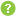0 = \$0.00 1 = \$21.95 2 = \$43.90 3 = \$65.85 4 = \$87.80 5 = \$109.75 6 = \$131.70 7 = \$153.65 8 = \$175.60 9 = \$197.55 10 = \$219.50 11 = \$241.45 12 = \$263.40 13 = \$285.35 14 = \$307.30 15 = \$329.25 16 = \$351.20 17 = \$373.15 18 = \$395.10 19 = \$417.05 20 = \$439.00 21 = \$460.95 22 = \$482.90 23 = \$504.85 24 = \$526.80 +MORE Printing Process:Digital Label Ink
Item # D117

Description

## Details

These trendy Pink Camo stickers can be used to personalize your child's books, lunch bags, CDs, etc. or they can be used as address labels. They coordinate nicely with our Pink Camo Notes

• Size: 2 1/2" D
• Paper Color: As Shown
• Ink Color: Black
• Ink Type: Flat
• Set includes 24 round stickers
• Reviews

Be the first to review this product

### You're reviewing:

Pink Camo Round Stickers

#### How do you rate this product? *

 1 star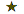2 stars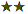3 stars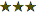4 stars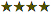5 stars